# Geology 56406640 23 Oct 2020 Introduction to Seismology

• Slides: 11
Download presentation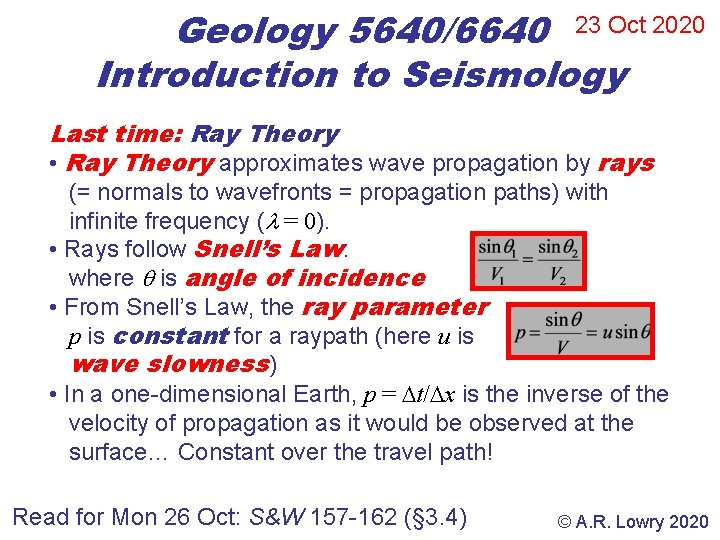Geology 5640/6640 23 Oct 2020 Introduction to Seismology Last time: Ray Theory • Ray Theory approximates wave propagation by rays (= normals to wavefronts = propagation paths) with infinite frequency ( = 0). • Rays follow Snell’s Law: where is angle of incidence • From Snell’s Law, the ray parameter p is constant for a raypath (here u is wave slowness) • In a one-dimensional Earth, p = t/ x is the inverse of the velocity of propagation as it would be observed at the surface… Constant over the travel path! Read for Mon 26 Oct: S&W 157 -162 (§ 3. 4) © A. R. Lowry 2020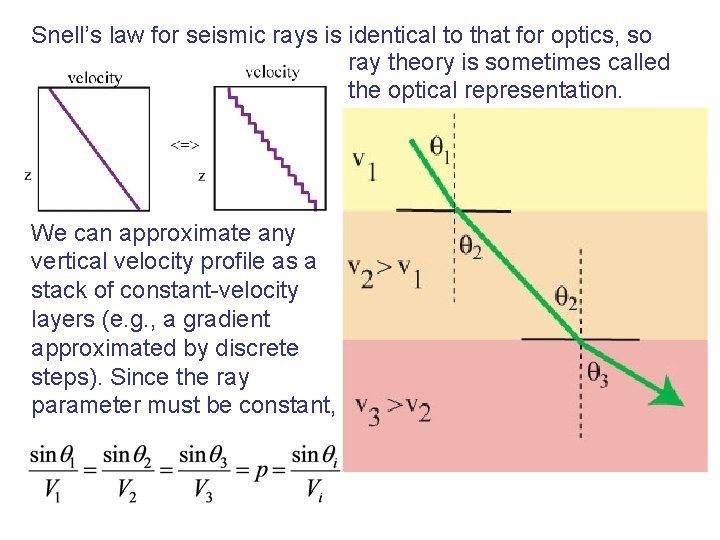Snell’s law for seismic rays is identical to that for optics, so ray theory is sometimes called the optical representation. We can approximate any vertical velocity profile as a stack of constant-velocity layers (e. g. , a gradient approximated by discrete steps). Since the ray parameter must be constant,If velocity continues to increase with depth, eventually one of two things happens. Either: 1) The ray follows the interface: sin i+1 = Or: 2) The ray reflects with no transmitted wave: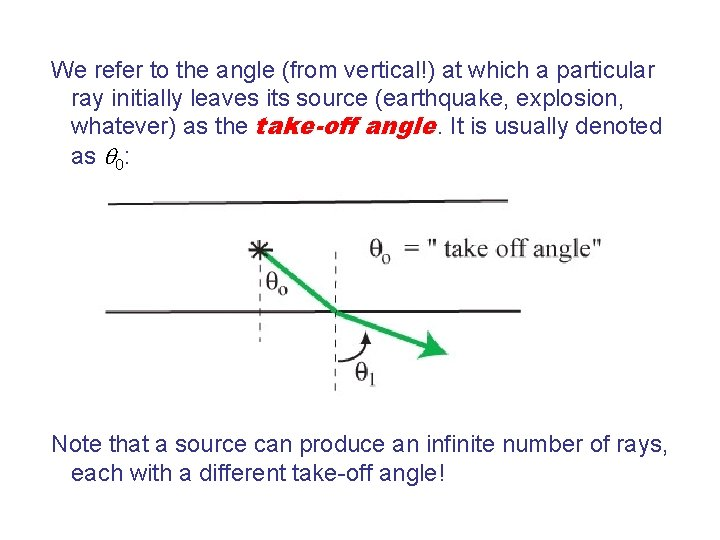We refer to the angle (from vertical!) at which a particular ray initially leaves its source (earthquake, explosion, whatever) as the take-off angle. It is usually denoted as : Note that a source can produce an infinite number of rays, each with a different take-off angle!For homogeneous, isotropic layers: 1) Ray parameter p is constant along a given ray path. 2) A smaller take-off angle translates to greater distance to where the ray path emerges at the surface (& a smaller ray parameter). 3) For a given velocity structure, every ray parameter maps to some distance ( ) from the starting point, and corresponding travel-time T = f(V).If we plot T vs. x (or )… Recall the plots of the seismic phases shown in previous lectures… The slope of the line gives the ray parameter for the phase arriving at that given ! Plots like this are common in seismology; called “T-X” or “T- ” or “time-distance” plots. Note the emphasis that is being placed here: The skeletalized information from the wavefield here is the travel-time; the information we are extracting about the Earth is velocity along the ray!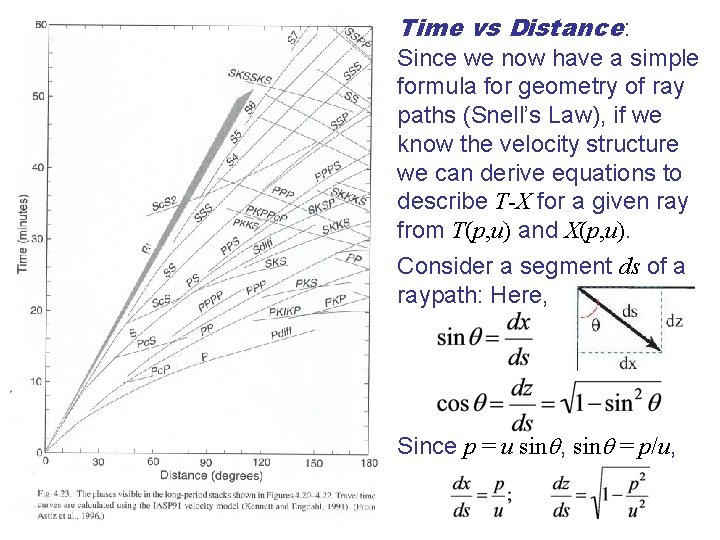Time vs Distance: Since we now have a simple formula for geometry of ray paths (Snell’s Law), if we know the velocity structure we can derive equations to describe T-X for a given ray from T(p, u) and X(p, u). Consider a segment ds of a raypath: Here, Since p = u sin , sin = p/u,Going the Distance… We can rearrange dz/ds as: Then using the chain rule: And plugging in our raypath relations: If we multiply both sides by dz and integrate: But p is a constant! SoIf we know u(z), we can integrate this from e. g. the surface down to a ray’s turning depth zp: And note that if we know u(z), we know zp: it’s the depth at which u ≤ p! Having solved for x(p), the distance X at which the ray will arrive at the surface is simply X = 2 x: Most often Earth velocity structure is represented as a layered stack (e. g. , PREM, which you’re looking at for HW). In that case the integral is a summation: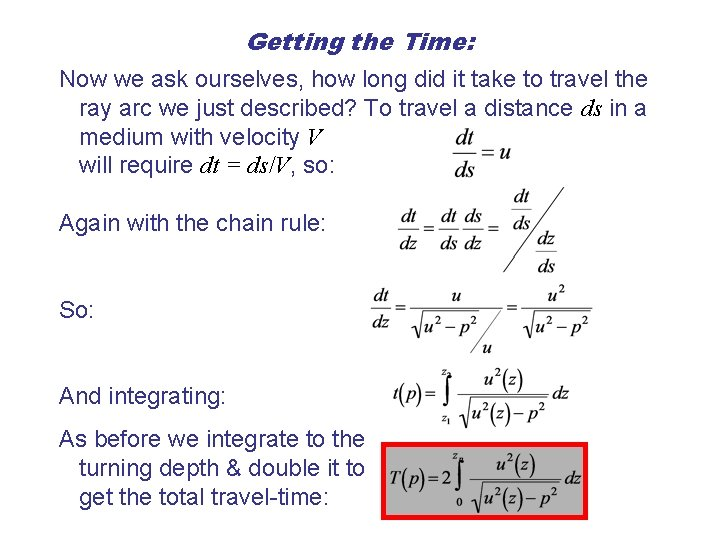Getting the Time: Now we ask ourselves, how long did it take to travel the ray arc we just described? To travel a distance ds in a medium with velocity V will require dt = ds/V, so: Again with the chain rule: So: And integrating: As before we integrate to the turning depth & double it to get the total travel-time: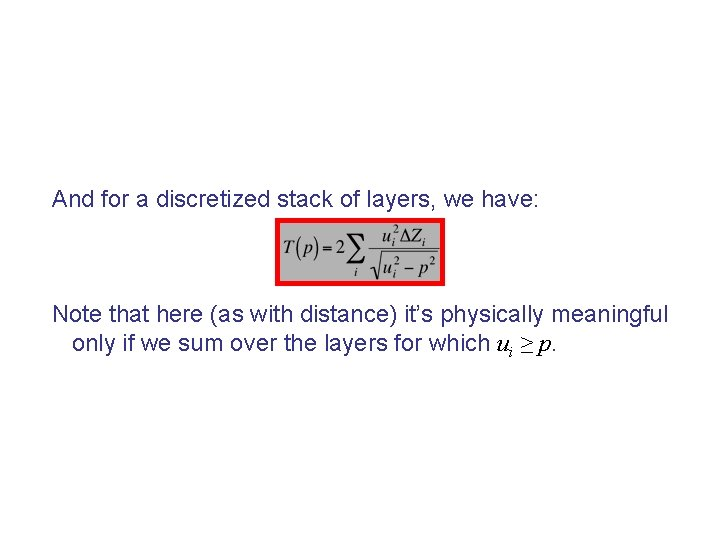And for a discretized stack of layers, we have: Note that here (as with distance) it’s physically meaningful only if we sum over the layers for which ui ≥ p.